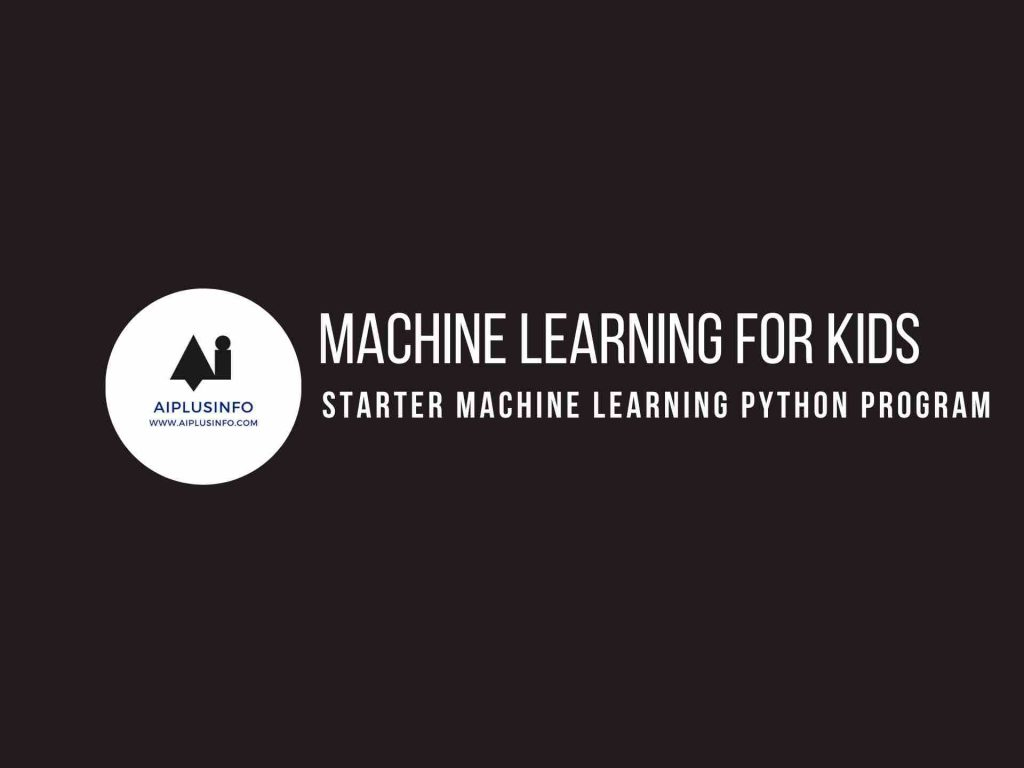# Machine Learning For Kids: Starter Machine Learning Python Program## Introduction

Welcome to this tutorial on Starter Machine Learning Python Program! Machine Learning is a method of data analysis that automates the building of analytical models. It’s a cool way to make computers learn from data and make predictions or decisions without being explicitly programmed to do so. In this tutorial, we’re going to write a simple machine learning program using Python.

## Who Is This For?

This tutorial is crafted for students in grades 6 to 10 who are already familiar with Python, including variables, data types, conditionals, loops, functions, and libraries. You should also have a basic understanding of mathematics. If you’re excited about stepping into the world of machine learning, then let’s dive in!

Also Read: How To Get Started With Machine Learning In Julia

## What Will We Learn?

Machine learning for kids: In this tutorial, we’ll learn how to create a simple machine learning model using a Python library called Scikit-learn. We will use the Iris dataset, which is a popular dataset in machine learning and statistics. It contains measurements of 150 iris flowers from three different species.

In machine learning, we usually have a dataset consisting of both input data and output data. The goal of a machine learning model is to learn a function that best maps the input data to the output data. This function can then be used to predict the output for new, unseen input data.

Scikit-learn is a Python library for machine learning that comes with many built-in datasets, like the Iris dataset, and tools for data processing, model creation, model training, and evaluation.

Here’s a basic machine learning program that uses the Iris dataset:

``````# Import the necessary libraries
from sklearn.model_selection import train_test_split
from sklearn.neighbors import KNeighborsClassifier
from sklearn import metrics

# Create features and target variable
X = iris.data   # features
y = iris.target  # target variable (species)

# Split dataset into training set and test set
X_train, X_test, y_train, y_test = train_test_split(X, y, test_size=0.2, random_state=4)

# Create KNN Classifier
knn = KNeighborsClassifier(n_neighbors=5)

# Train the model using the training sets
knn.fit(X_train, y_train)

# Predict the response for test dataset
y_pred = knn.predict(X_test)

# Model Accuracy
print("Accuracy:", metrics.accuracy_score(y_test, y_pred))``````

Here’s what the code does:

We start by importing the necessary libraries.

We load the Iris dataset with the `load_iris()` function.

`X` and `y` are our features and target variable, respectively. The features are the measurements of the flowers, and the target variable is the species of the flowers.

We split the dataset into a training set and a test set. The model will learn from the training set and we’ll use the test set to evaluate the model.

We create a K-Nearest Neighbors (KNN) classifier. KNN is a simple machine learning algorithm that classifies a data point based on the majority of its ‘k’ neighbors.

We train our KNN classifier using the `fit` method, and then we make predictions on the test set using the `predict` method.

Finally, we print the accuracy of our model. The accuracy is the proportion of the test set that our model classified correctly.

This program is a great start to your machine learning journey. You’ve learned how to create and evaluate a simple machine learning model in Python. In future tutorials, we’ll dive deeper into more complex aspects of machine learning. Keep exploring and happy coding!

## NEXT TUTORIALS

Tutorial 1 – Installing Python
Tutorial 2 – Your First Program in Python
Tutorial 3 – Python Variables
Tutorial 4 – Python Data Types
Tutorial 5 – Python Conditionals
Tutorial 6 – Python Loops
Tutorial 7 – Python Functions
Tutorial 8 – Advanced Python Functions
Tutorial 9 – Starter Machine Learning Python Program
Tutorial 10 – Your First Machine Learning Program!

Explore more with this book.

Python for Kids, 2nd Edition: A Playful Introduction to Programming
\$22.99
We earn a commission if you make a purchase, at no additional cost to you.
09/17/2023 12:41 am GMT

## References

Briggs, Jason R. Python for Kids: A Playful Introduction To Programming. No Starch Press, 2012.